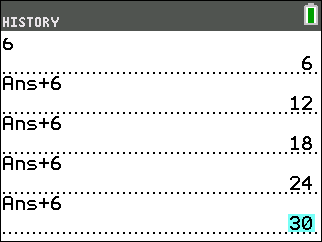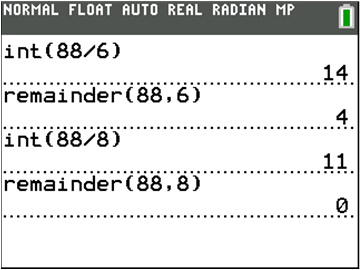••• ##### Device
• TI-83 Plus Family
• TI-84 Plus
• TI-84 Plus Silver Edition
•TI-84 Plus C Silver Edition
•TI-84 Plus CE

TI Connect™

• ##### Report an Issue

Middle Grades Math: Using Venn Diagrams to Find the GCD
by Texas Instruments#### Overview

In this activity, students use Venn Diagrams to classify numbers. They will first classify multiples of 3, 5, and 6, deciding where to place the least common multiple. Then, students will use the TI-84 Plus C Silver Edition™ integer division and remainder features to help them classify factors of three different numbers.

#### Key Steps

•In this activity, students use Venn diagrams to sort data. Specifically, students use the diagrams to analyze the factors and multiples of different numbers.  In the screenshot, the constant is +6.

•Students use the lcm( and gcd( features to investigate the number relationships in the Venn diagrams. Also, to find the factors of the three numbers given, students can use the integer division and remainder features to show the actual remainder from the division.